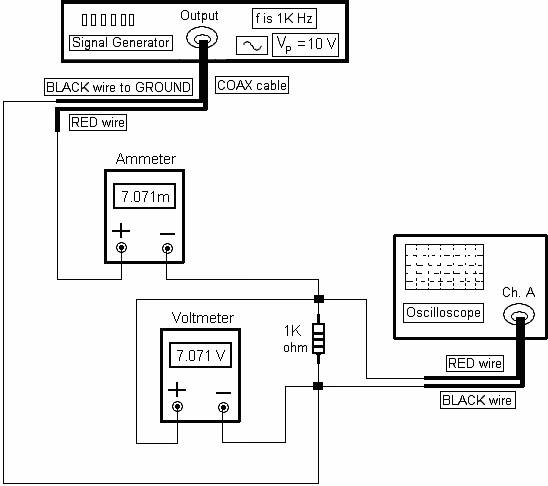RESISTIVE CIRCUITS (R-CIRCUITS): Figure 2.3 shows a resistive circuit.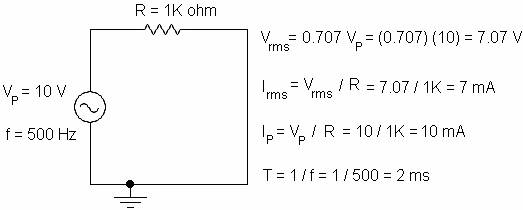Figure 2.3

Figure 2.4 shows the input voltage waveform of figure 2.3.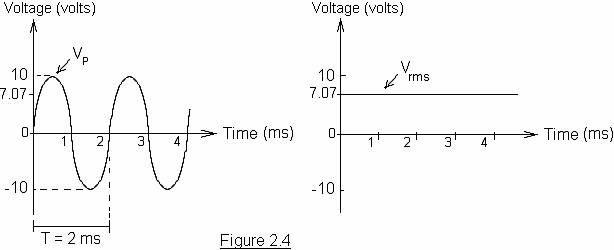Figure 2.5 shows the input voltage waveform is in phase with the current I of figure 2.3.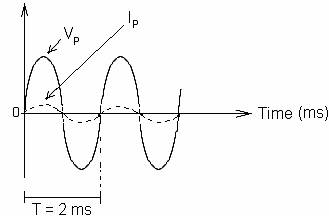Figure 2.5

Lab 2.1    Part One

Set up the circuit (software).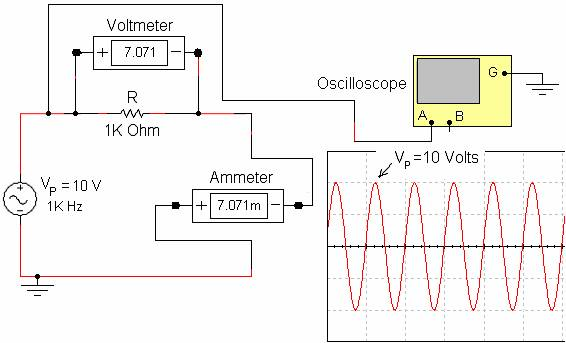In this circuit diagram, note the following:

VP = 10 volts,   f = 1K Hz,   T = (1 / f) = (1 / 1K) = 1 ms.

The voltmeter displays the RMS value of the voltage across the resistor R.

The ammeter displays the RMS value of the current in the circuit.

IP = VP / R = 10 / 1K = 10 mA.

Irms = (0.707) (10m) = 7.071 mA.

VR (rms) = Irms R = (7.071m) (1K) = 7.071 V.

Lab 2.1    Part Two

Set up the circuit diagram on page 9 using the following parts:Signal GeneratorOne 1K Ohm Resistor (1/4 W)Wire, 22 GaugeOscilloscopeTwo BNC-to-IC hooks (1 red and 1 black)One VoltmeterOne Ammeter